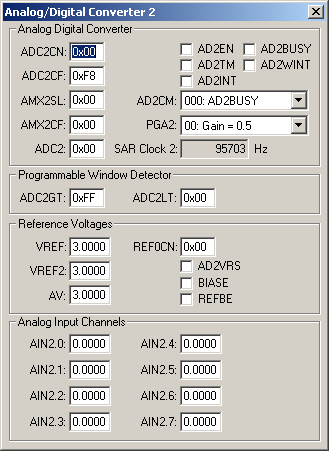Products Download Events Support Videos All Product Families ARM7, ARM9, and Cortex-M3 Products C16x, XC16x, and ST10 Products C251 and 80C251 Products Cx51 and 8051 Products Modified Anytime In the Last 7 Days In the Last 14 Days In the Last 30 Days In the Last 90 Days In the Last 6 Months

# Peripheral Simulation

For Silicon Laboratories, Inc. C8051F125 — A/D Converter (8x8-bit) + PGA

Simulation support for this peripheral or feature is comprised of:

• Dialog boxes which display and allow you to change peripheral configuration.
• VTREGs (Virtual Target Registers) which support I/O with the peripheral.

These simulation capabilities are described below.

### Analog/Digital Converter 2 DialogThe Analog/Digital Converter (ADC) dialog displays and configures the A/D converter. The controls in this dialog are separated into several logical groups.

Analog Digital Converter

• ADC2CN contains the following ADC control register settings:
• ADC2EN (ADC2 Enable) is set to enable the A/D converter.
• ADC2TM (ADC Track Mode) is set to enable low-power track and hold mode.
• ADC2INT (ADC Interrupt) is set when an A/D conversion completes.
• ADC2BUSY (ADC Busy) is set when and A/D conversion is in progress.
• ADC2WINT (ADC Window Compare Interrupt) is set when a Window Comparison Match occurs.
• ADC2CF contains the SAR Clock and PGA ADC control register settings.
• AMX2SL (Channel Select Register) is used to select the ADC input.
• ADC2CM (ADC Conversion Mode) selects the event that starts the A/D conversion.
• AMX2CF (AMUX2 Configuration Register) configures the analog input bit pairs for differential (bit = 1) or single-ended (bit = 0). Only bits 0-3 are used.
• PGA2 (ADC Internal Amplifier Gain) selects the gain value for the internal amplifier (PGA).
• ADC2 (ADC Data Byte) contains the 8-bit ADC result.
• SAR Clock 2 displays the calculated SAR (Successive Approximation Register) clock value derived from the system clock and bits 7-3 in ADC2CF.

Programmable Window Detector This feature continuously compares the output of the ADC to the following limit registers and notifies the system when an ADC output falls outside of this range:

• ADC2GT (Greater Than Data High Byte Register) is the high limit reference value.
• ADC2LT (Less Than Data High Byte Register) is the low limit reference value.

Reference Voltages

• VREF contains the bandgap output reference voltage.
• VREF2 contains the voltage at the ADC voltage reference input pin.
• AV (Analog Voltage) displays the analog supply voltage from the AV+ pin.
• REF0CN (Reference Control Register) contains the following controls:
• AD2VRS (ADC Voltage Reference Select) is set to use the AV output voltage in place of the VREF0 voltage for VREF.
• TEMPE (Temperature Sensor Enable) is set to enable the internal temperature sensor.
• BIASE (Bias Generator Enable) is set to enable internal bias voltage generation.
• REFBE (Reference Buffer Enable) is set to use the VREF pin to drive the internal voltage reference.

• AIN2.x (Analog Input Channels x) displays the analog voltage value input for each channel.

### AINx VTREGData Type: float

The AINx VTREGs set the analog input voltages for simulated A/D converters. The AINx VTREGs represent the inputs to the analog input pins of the MCU for analog input 0, 1, 2, and so on. AIN0 sets the input voltage for analog input #0, AIN1 sets the input voltage for analog input #1, etc. If you have properly configured the analog inputs, the following commands (typed in the debugger's Command Window) input the voltages specified.

```AIN0 = 0.000  /* Analog Input 0 = 0.000 volts */
AIN1 = 2.500  /* Analog Input 1 = 2.500 volts */
AIN2 = 4.999  /* Analog Input 2 = 4.999 volts */
```

You may create a debugger signal function to periodically change the value of the A/D input. The following signal function increases the A/D Channel 1 input voltage by 0.1 volts each second.

```signal void AIN1_sig (void) {
float f;

for (f = 0.0; f < 5.0; f += 0.1)
{
swatch (1.0);                  // Delay 1 second
AIN1 = f;                      // Set AIN1 Voltage
}
}
```

### AV VTREGData Type: float

AV is the analog supply voltage. Typing the following command in the debugger's Command Window sets the analog voltage to 3.0 volts:

```AV = 3.0
```

### SARCLK2 VTREGData Type: unsigned long

The Successive Approximation Register (SAR) clock used for A/D conversion. It is based on the system clock (CLOCK VTREG) and can be adjusted by the setting in bits 7-3 of ADC2CF.

### VREF VTREGData Type: float

VREF contains the bandgap output reference voltage.

### VREF2 VTREGData Type: float

VREF2 is an input pin that provides a voltage reference input for ADC2. The AD2VRS bit in the REF2CN control register enables it's use with the ADC.